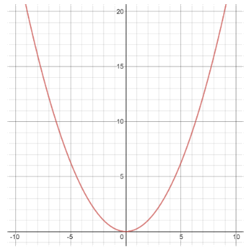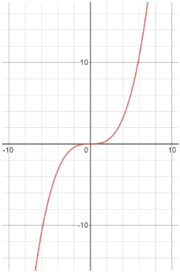# Even and odd functions

Even and odd are terms used to describe the symmetry of a function. An even function is symmetric about the y-axis of a graph. An odd function is symmetric about the origin (0,0) of a graph. This means that if you rotate an odd function 180° around the origin, you will have the same function you started with.

Most functions will be neither even nor odd. The only function that is even and odd is f(x) = 0.

To see if a function is even, you can imagine folding the graph along its y-axis. If the function has folded onto itself, then it is even.

To see if a function is odd, you can imagine folding the graph along its y-axis then along its x-axis (or vice versa). If the function has folded onto itself, then it is odd.evenodd

One way to remember the difference between even and odd functions is to remember that both "odd" and "origin" start with the letter "o." Then you just need to remember that the other type of function, even functions, are symmetric about the y-axis.

You will not always have a graph of the function at your disposal, so sometimes you must prove that a function is even or odd (or netiher) using algebra.

### All even functions follow the rule:

f(x) = f(-x)

This means that each x value and -x value have the same y value.

Thus, to see if a function is even, plug -x into x and simplify. If the resulting function is the same as the original, the function is even.

Example

f(x) = 5x4 + 4x2 + 2

5(-x)4 + 4(-x)2 + 2

f(x) = 5x4 + 4x2 + 2

f(-x) = f(x)

### All odd functions follow the rule:

f(-x) = -f(x)

This means that each x value has a y value that is the opposite of the y value of their corresponding -x value.

To see if a function is odd, plug -x into x and simplify. If the resulting function is the original function multiplied by -1, then the function is odd.

Example

f(x) = 5x5 - 4x3 + 2x

f(-x) = 5(-x)5 - 4(-x)3 + 2(-x)

-5x5 + 4x3 - 2x = -(5x5 - 4x3 + 2x)

f(-x) = -f(x)

If the resulting function does not follow either rule, the function is neither even nor odd.

You may have noticed that even functions only have even exponents, and odd functions only have odd exponents. This is true for polynomials, but there are many rational and trigonometric functions that are even or odd as well.